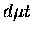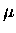Next: Hyperspherical approach Up: Variational approaches Previous: Hylleraas type basis expansion

### Coupled rearrangement channel method

A very appealing method in calculating three body problems, known as the non-adiabatic coupled rearrangement channel method , was developed by Kamimura. The method has been applied to many muonic and other three-body problems [112,113,114,48,115,44,47,45,46]. In his approach, the total three body-wave function is expanded in basis functions spanned over three rearrangement channels in the Jacobian co-ordinate system, which is illustrated in Fig. 2.2 for the case of thesystem.The method treats the muon on an equal footing with the other two nuclei. All three channels are explicitly employed with each having its own importance; since the (J,v)=(1,1) state lies only0.66 eV below the-d (c=1 in Fig 2.2) break up threshold, and48 eV below the-t (c=2) threshold, the large components of the three body wave functions are expected to be in the c=1 and c=2 configurations. The [dt]-component (c=3) is important for calculations of fusion and sticking where the details of the wave functions at small r3 are needed.

Gaussian gemials are used as basis set functions to describe radial dependences of the wave function. For each channel c, the basis has the form==(35)

whereandare the nonlinear parameters to be optimized, and lc, Lc stand for the relative angular momentum associated with co-ordinatesrespectively. The use of a Gaussian basis allowed straightforward analytical integrations in calculations of kinetic and potential energy matrix elements. Some detailed examples are given in Ref. . A faster damping'' of the Gaussian tail, compared to the Hylleraas type functions with exponential tails, is not a problem even when representing a diffuse (1,1) state, since the parametersandcan be made much larger than the muonic molecular size.

In addition to the philosophical appeal of treating the muon on the same footing as the nuclei, as mentioned above, this method possesses some practical advantages, which include the following. First, because the basis spans three channels, linear dependencies among the basis functions is smaller, i.e. non-orthogonality is not too severe, compared to the single-channel basis function. This permitted calculations in double precision (64 bits, 1416 decimal digits), which resulted in a very short computation time. Second, due to the explicit use of Jacobian co-ordinates with the rearrangement channels, the method is suitable for scattering calculations, where correct boundary conditions are satisfied (c.f. recall the problems with the Adiabatic Representation). This feature is also useful in describing thewave function in the calculation of molecular formation rates (see Section 2.2).

The relative contributions of each channel can be tested with this method by removing one channel from the calculations, which proves the relative importance of the channels, in the order (c=1) > (c=2) >(c=3), as expected above, but also shows that to achieve the 10-3eV level of accuracy in, it is necessary to include c=3. With the basis set of, the final accuracy of abouteV was achieved, which includes the estimated uncertainty in extrapolation to.

Among other approaches for the three-body Coulomb problem, two of them, which have received recent attention inCF theory, are briefly discussed below.Next: Hyperspherical approach Up: Variational approaches Previous: Hylleraas type basis expansion